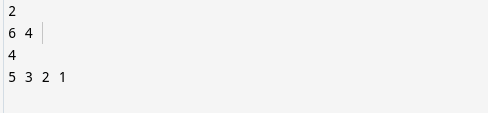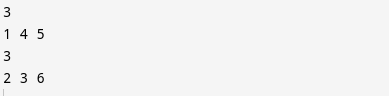# Divide 1 to n into two groups with a minimum sum difference

## Question

Given a positive integer n, n > 2. The task is to divide numbers from 1 to n in two groups such that the absolute difference of the sum of each group is minimum.

Input: An integer n.

Output: Print the size of any of the two groups in the first line, then print the elements of that group in the second line. Similarly, print the size of the remaining group in the next line and then its elements.

The questions you should ask the interviewer:

1. What is the constraint on n?
2. If there are multiple groups possible, how to give output in that case?

Examples:

Alert Ninjas: Don't stop now! Enroll in the Competitive Programming course today and start coding. Learn the art of writing time and space-efficient code and compete in code competitions worldwide.

Recommended: Please try to solve it yourself before moving on to the solution.

## Solution:

#### Approach 1:

Idea: The idea is to use the fact that we can always divide 1 to n into two groups such that the absolute minimum difference of the sum is either 0 or 1. As the sum of all 'N' elements will be either odd or even. So partition will be,

• if the total sum is odd => odd = odd + even, in this case, the absolute minimum difference can be 1.
• if the total sum is even => even = o+o / e+ e, in this case, the absolute minimum difference can be 0.

Implementation

1. Consider two groups Group1 and Group2.
2. We define a variable halfSum as half of the sum of n components.
3. Now, in a loop from n to 1, enter i into group1 if the sum of the elements in group1 does not exceed halfSum; otherwise, insert that i into group2.

C++

Output:Time Complexity: O(N) as we are only using a for loop from range 1 to n, which uses O(N) time.

Space Complexity: The space complexity is O(N) as we are using extra space to create two groups of maximum size n.

#### Approach2:

Idea: As the elements are consecutive, we can always say that in a series of four continuous elements, the sum of extremes is always equal to the sum of middle elements.

Example:

1 2 3 4

1+4 = 2+3 = 5

5 6 7 8

5+8 = 6+7 = 13

And so on…

Implementation:

1. Check if n is odd or even.
2. If odd, divide the elements 1 to n in pairs. E.g., add (1,2) in group1, (3,4) in group2, then (5,6) in group1, and so on.
3. If even, consider the groups of 4. Add the extremes to one group and the central elements to the other group. There can be a chance that two elements remain in the end; add one-one element to each group.

C++

Output:Time Complexity: O(N)

Space Complexity: The space complexity is O(N) as we are using extra space to create two groups of maximum size n.

## FAQs

1. What are important math topics required for interview preparation?
Some important maths concepts a programmer should focus on includes Mathematical Induction, Calculus, Probability, and Statistics, Graph Theory, etc.

2. What is array partitioning?
In a given array of objects, in random order.  You want to split the array in half, with the lower half containing objects that match the condition and the top half containing things that do not. This is called the partitioning of an array.

## Key Takeaways

Today we solved one mathematical coding question: divide 1 to n into two groups with a minimum sum difference with two approaches. In Approach 1, we solved by half sum calculation, and in the 2nd approach, we used the fact that in a series of four continuous numbers, the sum of extremes equals the sum of the middle elements.

Apart from that, you can use CodeStudio to practice a wide range of DSA questions asked in lots of interviews. It will assist you in mastering efficient coding techniques, and you will also get interview experiences from people working in big companies.